# Webinar «What Do We Need to Calculate to Solve the Issue of Electromagnetic Compatibility with the Lightning Current?», page 2

## Webinar text. Page 2

– Now, what can we do with the underground utility? We can do the same as with an air utility. We can take the utility with its inductance and replace it with the leakage resistance because the utility is in the earth and the current flows from it into the earth all the time. But such replacement provides a solution that is far from the real situation because when the lightning current goes to the utility, this leakage first effects at the length of 1 cm, then 1 m, and then 10 m, 20 m, and so on, i.e. it turns out that the inductance and the resistance are distributed in space. Then, the equation that should be written here, must be provided using the partial derivatives for the line with distributed parameters. And this solution cannot be obtained that easy. This task must be solved using a computational method by solving an equation with the partial derivatives on a PC. And I have showed you this solution that was obtained for the utility of 100 meters long that is located in the soil with the resistivity of 100 Ohm*m, and it has got the earthing resistance of 10 Ohm at the one end and 1 Ohm at the other end. And you can see that the current in the underground utility is not 17% as it is shown in GOST, but almost 40%. Alternatively, it may even reach 50 or 60%. What can we do in this case? You know, you cannot replace the solution of equation using the partial derivatives that easy as I have proposed for the air line. What do we ensure? We ensure the following thing: in the book that is being published by the ZANDZ Project, you will have a chance to choose among three software applications that will be available for the owners of the book. These applications will be installed on the company's website, and the book will contain the information on how to access them free of charge. And we provide the applications free of charge.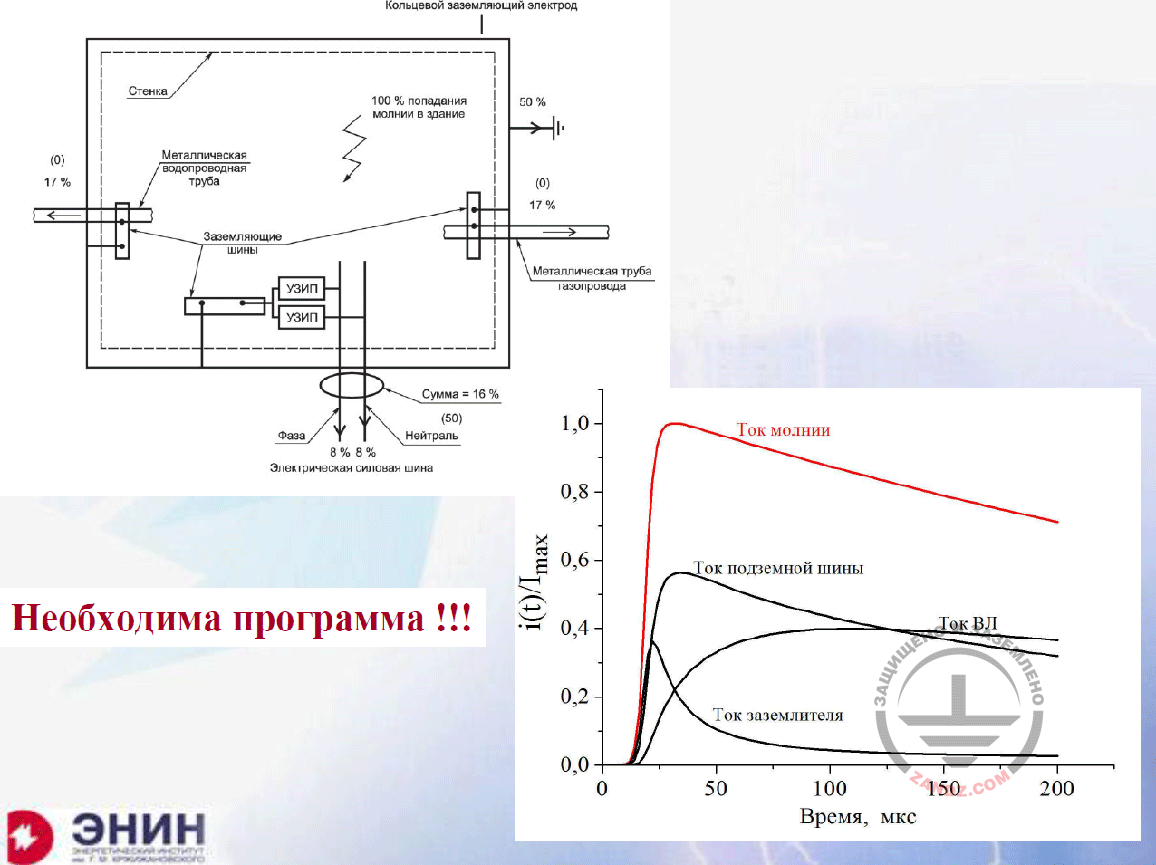We need a software!

Кольцевой заземляющий электрод
Стенка
Металлическая водопроводная труба
Заземляющие шины
УЗИП
Фаза
Электрическая силовая шина
Нейтраль
Сумма
Металлическая труба газопровода
Ток молнии
Ток подземной шины
Ток ВЛ
Ток заземлителя
Время, мкс
Необходима программа!

Ring earthing electrode
Wall
Metal water supply pipe
Earthing buses
SDP
Phase
Electrical power bus
Neutral wire
Sum
Metal pipe of a gas pipeline
Lightning current
Underground bus current
Power line current
Earthing device current
Time, mcs
We need a software!

You will be able to solve this task, if you need it free of charge. And the task you must solve instead of the one shown here (taken from GOST), where 17% of the current flow along the utility and 50% go to the earthing device. You can see the solution I have provided as an example. 40% of the current instead of 16% entered the line, and 58% instead of 17% entered the underground utility, while about 38% got into the drain of the earthing device of the facility, and it decreased rapidly. This is the situation that takes place rather than the mess provided by the typical GOST solution. And certainly, for any real-life utilities, you may obtain such calculated curves for the case in interest using these applications. When I wrote the book, the company's management provided guarantees for me that you will have such opportunity.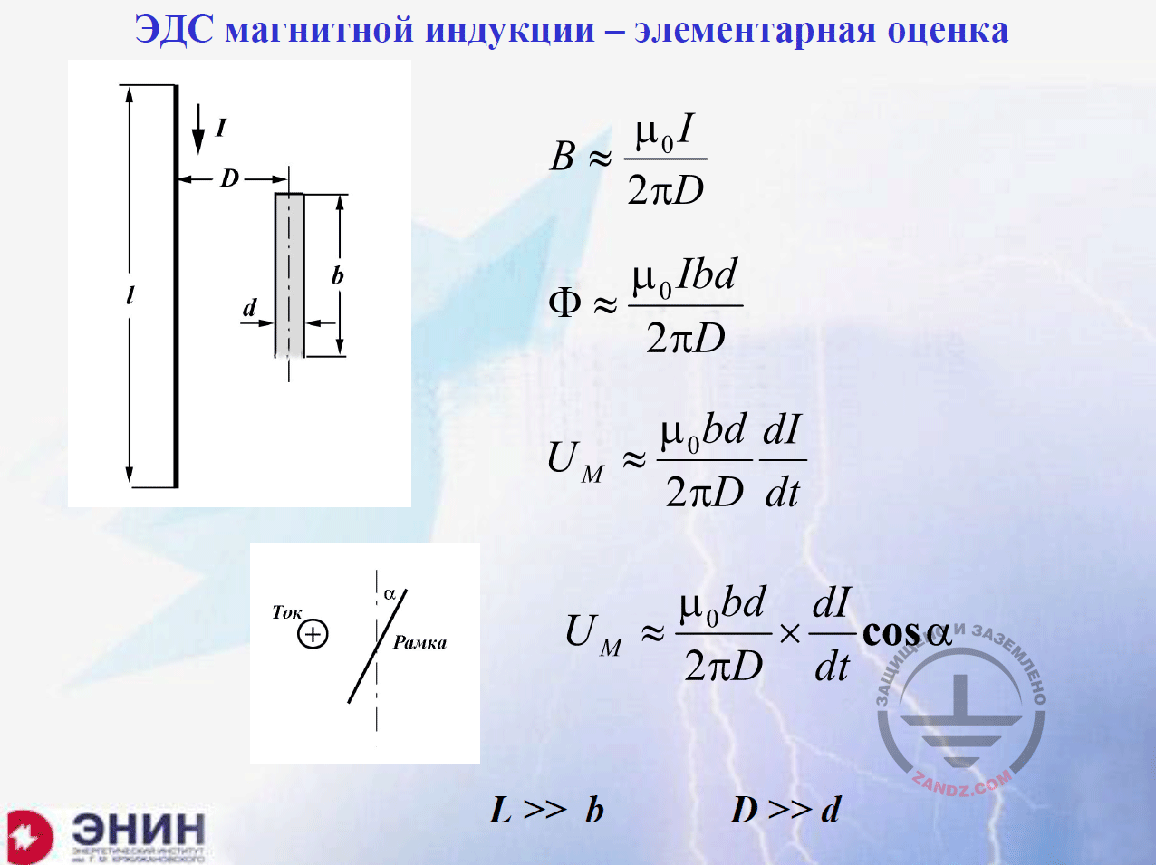Electromotive force of magnetic induction: a simple estimate

ЭДС электромагнитной индукции – элементарная оценка
Ток
Рамка

Electromotive force of magnetic induction: a simple estimate
Current
Frame

Now, let's go further to the induced surges. Electromagnetic force of magnetic induction: what does GOST state? GOSTs provide a simple thing. GOSTs provide that the magnetic induction from the current is determined by the magnetic permeability and the distance from the current to the circuit, for which the electromagnetic force of magnetic induction is calculated. This formula is used in all reference guides. By the way, it is also used in a simple physics textbook for secondary schools. And this formula is only true when you have got a circuit in consideration and it has got a width that is much less than this distance to the conductor with the current, and the length of this circuit is much shorter than the length of the conductor with the current. In other words, it is all true only for the infinitely long current source. And then, the electromotive force of magnetic induction can be simply determined using the current derivatives and two dimensions. One dimension is a length of circuits, for which the electromotive force of magnetic induction is calculated, and another dimension is a width of circuits, for which the electromotive force of magnetic induction is calculated. For example, such circuit may be a standard pair of conductors, a flat pair, that is placed onto the earth, hung on the wall or something like that. The question is: what happens if such pair is not laid in parallel as shown here but it is placed at the angle relative to the axis (at the angle α)? It is also obvious what happens.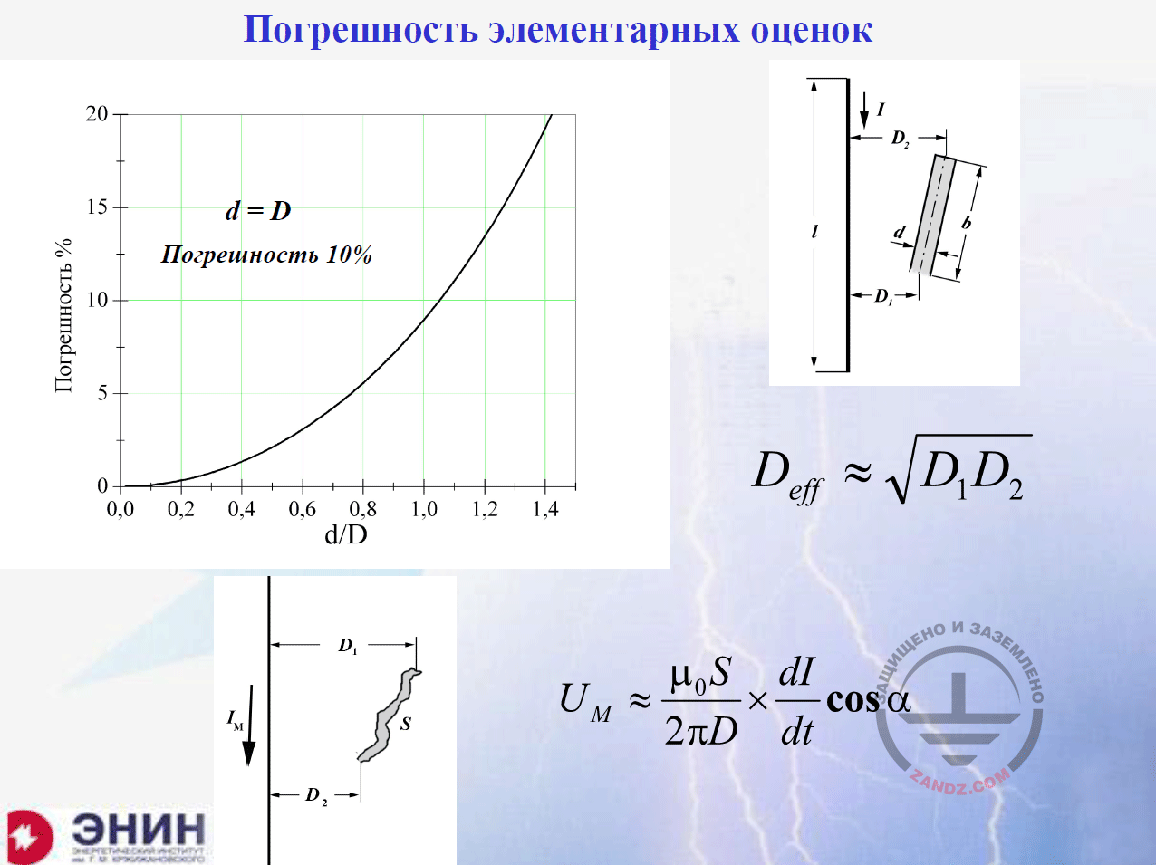Error of simple estimates

Погрешность элементарных оценок
Погрешность %
Погрешность 10%

Error of simple estimates
Error, %
Error 10%

It turns out that the formula is low sensitive to the dimension ratios of the distance to the circuit and the circuit width. Even if these two distances are equal, the error of an engineering calculation will be only 10%. Can we tolerate such error? I think, we surely can. Because the lightning current measurements are performed with high errors. Now, we have got one more question. If the situation is as follows: we have got the conductor with the current and the circuit, wherein the circuit is now not parallel to the conductor, it is angled, and we have got two different distances, can we use this simple, primitive formula? I think, there is no risk at all. We need to use the geometrical average of these distances as shown here instead of distances D1 and D2. And calmly use this formula. I assure you that your error will be within the acceptable ranges. And finally, the following question: what if the circuit is snake-shaped? I have drawn it here like this; it is curved differently. Again, we can use this formula, but instead of multiplying the length by the width, we have to use the cross-section of this circuit, S. If you use the distance S instead of multiplying the length by the width and use the geometrical average dimension, D, you will obtain the acceptable result. I assure you that nobody will have any doubts in your calculations as they are rather acceptable.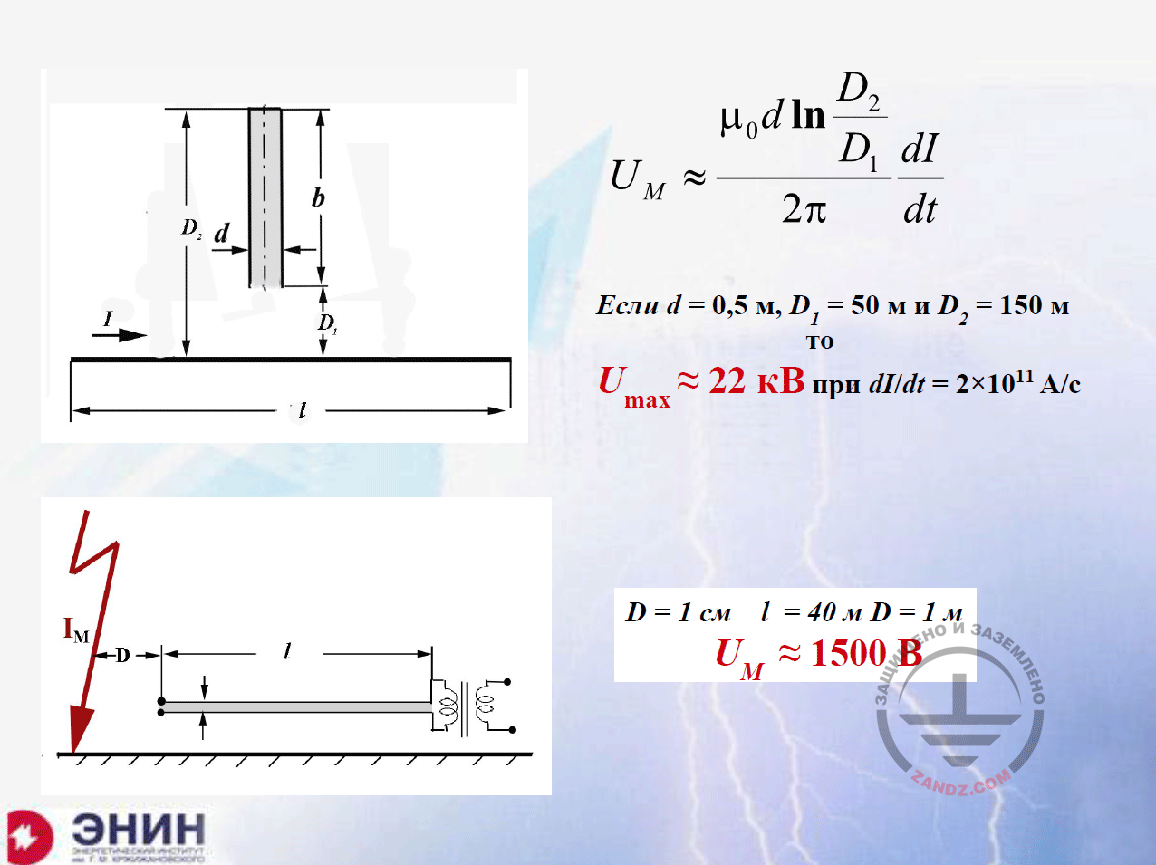Calculation formula

Если
то
при
кВ
А/с
м
см
В

If
then
at
kV
A/s
m
cm
V

Now, here is another variant we have to deal with. In this case, the conductor with the current is directed orthogonal to the circuit of interest. Rotate this picture in your mind by 90°. The current will flow along the lightning channel. And this circuit will be the power line that goes away from this circuit. And this is a real situation we have to consider. Then the simple calculation expression appears again, wherein the ratio between two distances of the first and the second are used along with the logarithm of these ratios, and it yields the electromotive force of magnetic inductance. For example, imagine that the lightning strike occurred at a distance of 50 meters from the power line with the standard air wires and the distance between the wires of about 50 cm; the length of this line is 100 meters. If you use the maximum calculation speed of the lightning current increase of 2 x 1011 A/s, the formula will provide an electromotive force of magnetic inductance equal to 22 kV, which burn everything that is connected to this line. I can say that the formula works correctly, because I have paid 5,000 RUB to repair such instruments when it happened near my house in the Moscow Region. And I want to show you another variant. An institute employee came to me and said: "Do you have the knowledge in lightning protection?" I said that I probably did. A person came to me: "The lightning struck my house!" I said: "Very good". "I had a charger plugged into the socket in the house, and the battery was charging in the garage. When the storm began, I turned off the socket, and the charger was turned off. The socket was on the earth". I said: "So what?" "The new expensive charger has burnt out!" Consider the situation: can the turned-off system burn out? Here is the lightning strike, the distance D of about one meter, the wire length to the garage was about 40 meters. If you use these values in this formula, then you will see that the circuit had the voltage of about 1.5 kV. What happened next? The next thing was: due to the poor insulation between the contacts in the plug, these 1.5 kV surpassed, and then all of them went to the primary winding of the transformer that generated the direct current to the battery powered from it.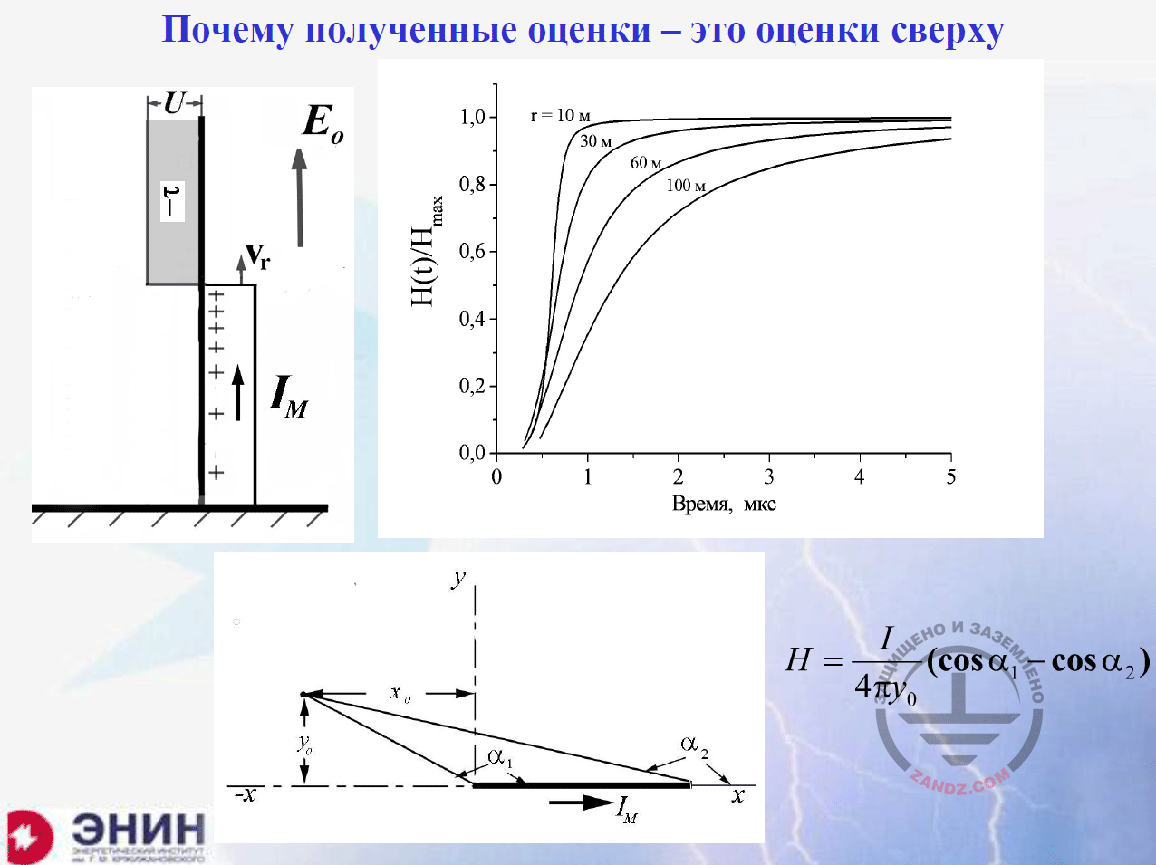Why are the estimates obtained from the top?

Почему полученные оценки – это оценки сверху
Время, мкс

Why are the estimates obtained from the top?
Time, mcs

The most important point is that all estimates I have shown to you for the electromotive force of magnetic inductance should be considered as the ones obtained from the top. They yield the overstated values of the electromotive force of induction. Why are they overstated? You need to understand that.

– Eduard Meerovich, I propose to stop at this point, make a pause, and answer a question. I think it is better to answer it right now.

– OK.

– Shamil asks: "Please tell us why do not you take into account the capacitive resistance? For example, when calculating the short-circuit current of the single-phase short circuit to the earth (we may conditionally assume that the lightning strike is similar to the single-phase contact to the earth when we bear in mind the distribution path)? So please tell us, how diverse is the harmonic component of the lightning and if we have to consider this in the inductive resistance?"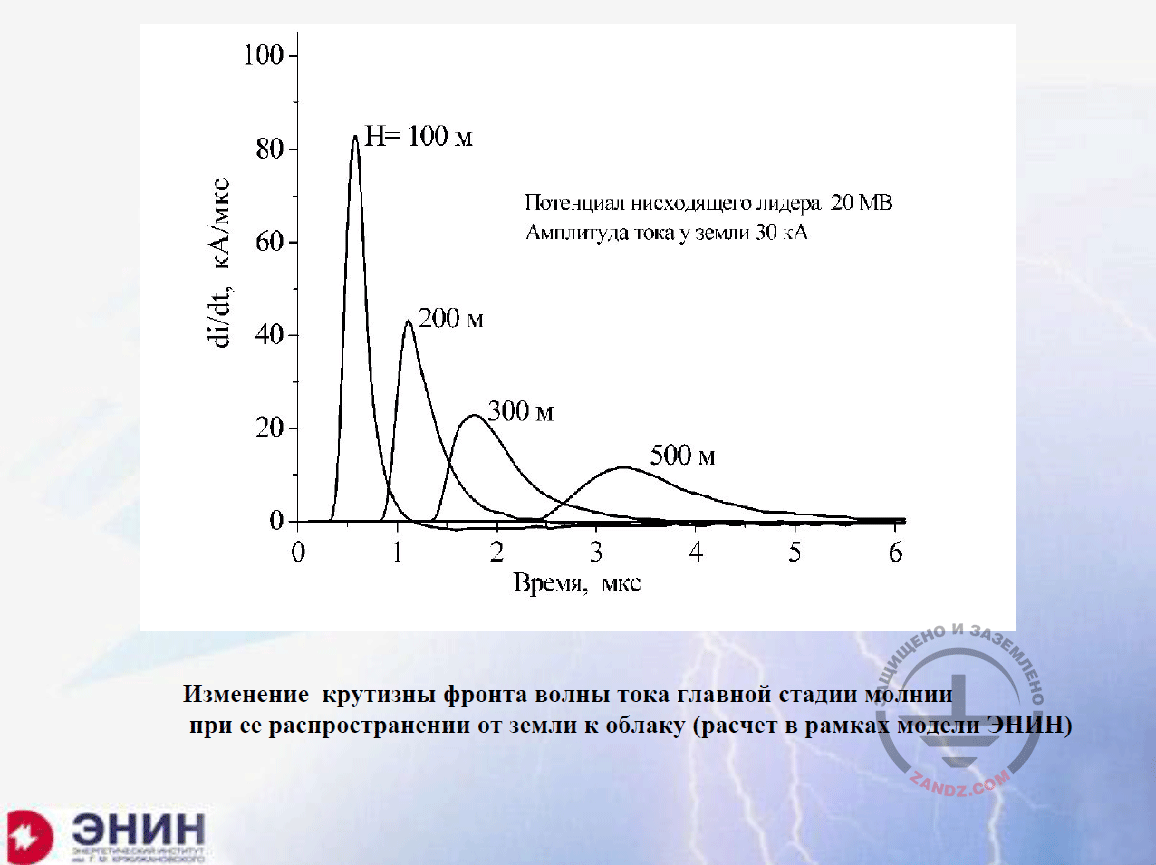Changes in the steepness of the lightning current wavefront

кА/мкс
Время, мкс
Потенциал нисходящего лидера 20 МВ
Амплитуда тока у земли 30 кА
Изменение крутизны фронта волны тока главной стадии молнии при ее распространении от земли к облаку (расчет в рамках модели ЭНИН)

kA/mcs
Time, mcs
Potential of a downward leader 20 MV
Current amplitude near the earth 30 kA
Changes in the steepness of the lightning current wavefront of the main stage of lightning upon its distribution from the earth to the cloud (calculation within the ENIN model)

The third factor that exists is the following one. When the current wave runs along the lightning channel, it runs along the conductor with a rather high resistance, because although this is a hot plasma, but it is a low-temperature plasma with the temperature of 20 to 30 thousand degrees, and its conductance is finite. Therefore, the wave that runs along the same plasma channel fades away gradually. The derivative of this wave at the distance of 100 meters had one value while at the distance of 500 meters it was different, and this is not considered in the simple formulas, again. Therefore, if you need to calculate with a very high accuracy, you need to take all of these into account. We would have to take them into account if we precisely knew the lightning current parameters and this running wave parameters, but they are not known to us, they are inaccurate; therefore, such refinement is a nit-picking. It is better to estimate from the top and be sure in the devices you will install according to this estimate from the top. And I think this is the most important point.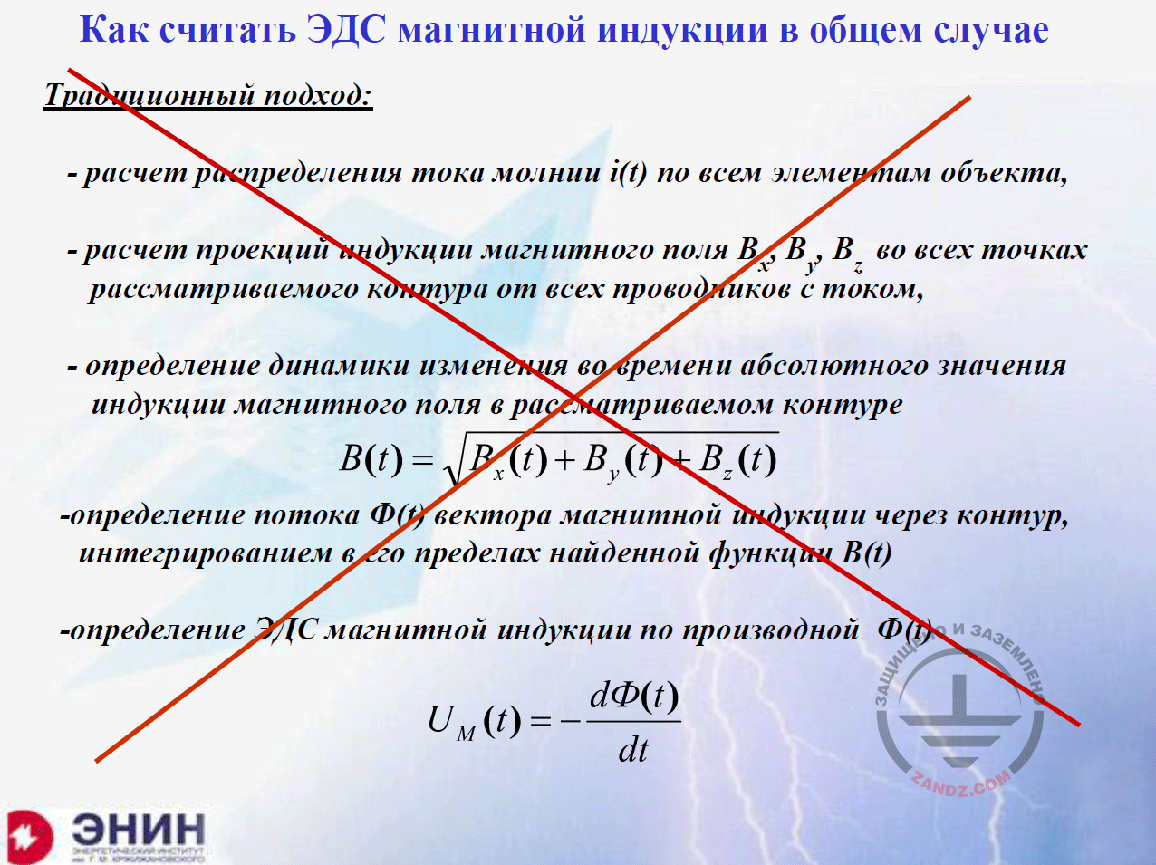How can we calculate the electromotive force of magnetic induction in a general case?

Как считать ЭДС магнитной индукции в общем случае
Традиционный подход:
- расчет распределения тока молнии i(t) по всем элементам объекта,
- расчет проекций индукции магнитного поля Bx, By, Bz во всех точках рассматриваемого контура от всех проводников с током,
- определение динамики изменения во времени абсолютного значения индукции магнитного поля в рассматриваемом контуре
- определение потока Ф(t) вектора магнитной индукции через контур, интегрированием в его пределах найденной функции B(t)
- определение ЭДС магнитной индукции по производной Ф(t)

How can we calculate the electromotive force of magnetic induction in a general case?
- calculation of the lightning current i(t) distribution among all facility elements,
- calculation of projections of the magnetic field Bx, By, Bz induction in all points of the considered circuit from all conductors with the current,
- determination of the dynamics of changing over time of the absolute value of induction of the magnetic field in the considered circuit
- determination of flow Ф(t) of the vector of magnetic induction through the circuit, integrating it within the determined function B(t)
- determination of the electromotive force of magnetic induction by derivative Ф(t)

Now, I want to show you one more thing that I believe to be very important for us. It is considered in the software applications we offer in the book. Imagine that you are calculating the electromotive force of magnetic induction in the territory of a facility. You need to calculate currents in all conductors, calculate the magnetic field from each current, summarize them as vectors and further calculate the magnetic flux that goes across the circuit wherein the electromotive force of magnetic induction is estimated; determine this magnetic flux value, differentiate it according to the time, and after that you will obtain the electromotive force of magnetic induction.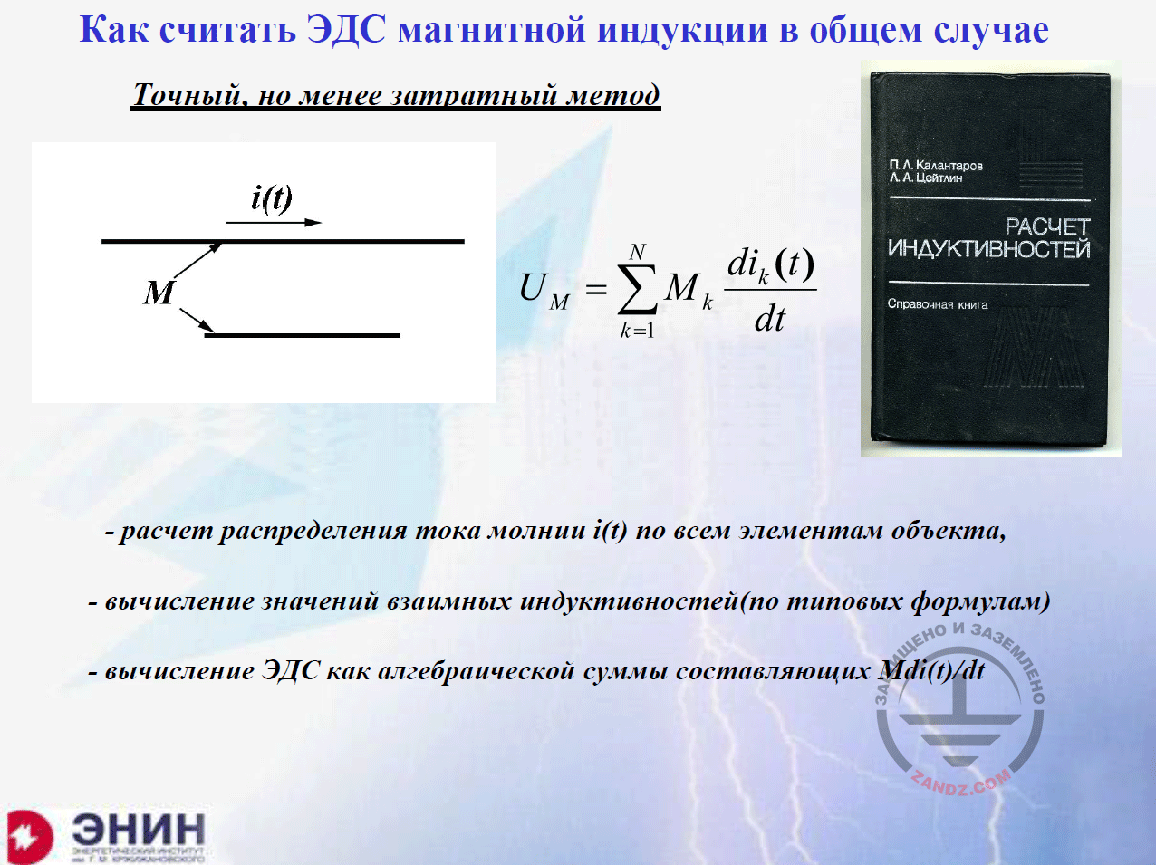How can we calculate the electromotive force of magnetic induction (another method)?

Как считать ЭДС магнитной индукции в общем случае
Точный, но менее затратный метод
РАСЧЕТ ИНДУКТИВНОСТЕЙ
- расчет распределения тока молнии i(t) по всем элементам объекта,
- вычисление значений взаимных индуктивностей (по типовым формулам)
- вычисление ЭДС как алгебраической суммы составляющих Mdi(t)/di

How can we calculate the electromotive force of magnetic induction in a general case?
Accurate but less expensive method
CALCULATION OF INDUCTANCES
- calculation of the lightning current i(t) distribution among all facility elements,
- calculation of mutual inductances (according to typical formulas)
- calculation of electromotive force as an algebraic sum of Mdi(t)/di components

I used this approach. I thought that we had to use it and developed a software application of this kind until a very formalized person who did not like the excessive efforts said the following: "What do you need it for? You do not need to do it!" Why? And he drew my attention to the following aspect: there are one conductor with the current and another conductor wherein the electromotive force of magnetic induction is induced by this current. How is it induced? If I know the mutual inductance between these conductors, the electromotive force of magnetic induction will be a mutual inductance multiplied by the current derivative over time. If there are many such conductors, then you have to find the electromagnetic force of mutual magnetic induction with all conductors. To do this, we have got a book for the inductances calculation, wherein all formulas for calculating the mutual inductances for differently arranged conductors are provided. Then, take these components, sum them up while considering the algebraic sign, and you will obtain the electromotive force that exists in a circuit of any shape. When we have developed a software application and compared two applications, we were surprised in that they provide the same result, but such calculation is the simplest, despite the fact that the calculations of some mutual inductances require complex formulas. To avoid using such formulas, we have created a software application that does not require calculating these inductances, and we offer it to you. You just input the required currents there and obtain the electromotive force of magnetic inductance in the form you need. This is what I would like you to use as a tool; and this work is worth doing it.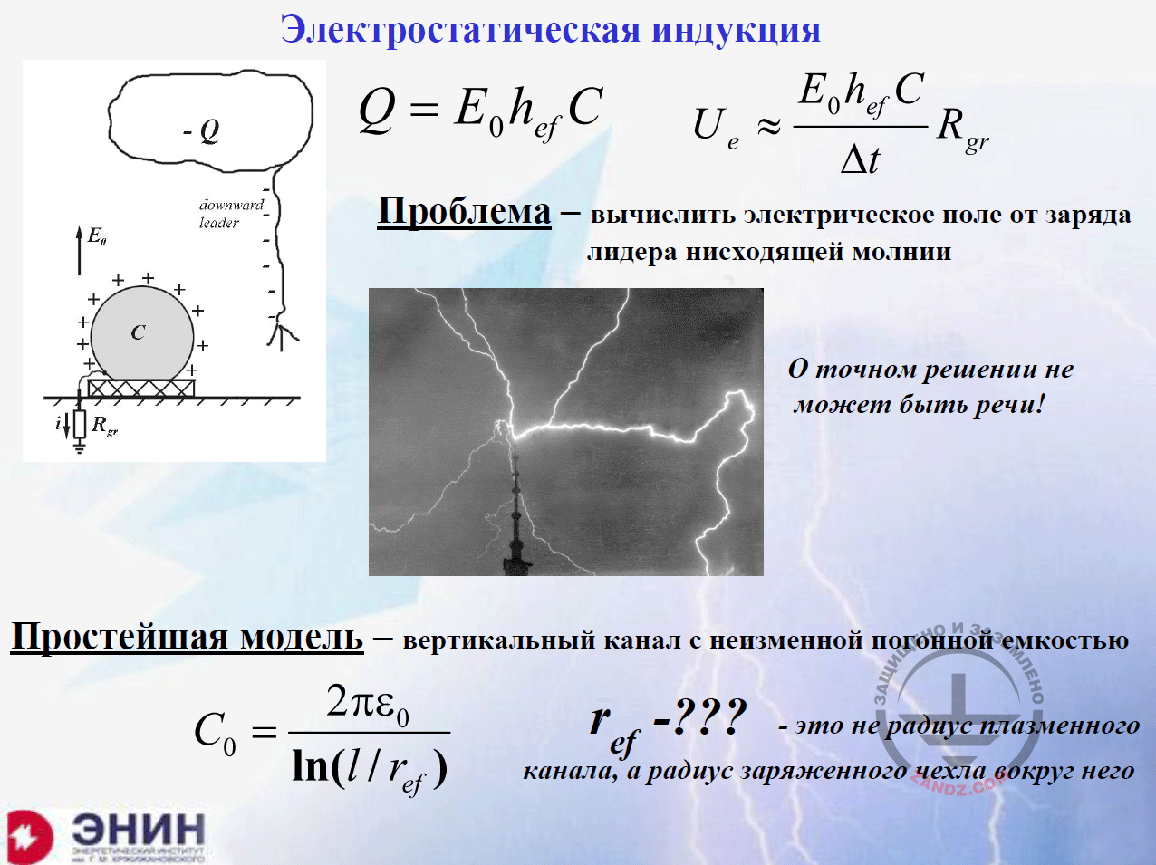Electrostatic induction

Электростатическая индукция
Проблема – вычислить электрическое поле от заряда лидера нисходящей молнии
О точном решении не может быть речи!
Простейшая модель – вертикальный канал с неизменной погонной ёмкостью
- это не радиус плазменного канала, а радиус заряженного чехла вокруг него

Electrostatic induction
The problem: to calculate the electrical field from the downward lightning leader charge
Accurate solution is out of the question!
The simplest model is the vertical channel with the permanent capacity
- is not the plasma channel radius but the radius of the charged enclosure around it

And finally, the last point, for which I have prepared all approaches, but I do not want to do it for the following reason: there is also an electromotive force of electrostatic induction, and I described it in the previous webinar. In the previous webinar, I said the following: if you have got an onground facility that has been earthed through the earthing resistance, and you have got a moving lightning channel nearby, then this lightning channel induces the charges due to the electrostatic induction. These discharges flow from the earth on the surface of such conductor. But due to the fact that the lightning channel moves slowly, the charges flow slowly and no surge occurs from this. The current that flows through the earthing device is low, but when the channel grows to the earth, its charge neutralizes, and it happens within microseconds, and the electric field disappears rapidly. And such induced charge flows rapidly into the earth, and the electromagnetic force of electrostatic induction is created. And now it is a significant current with the value of several kiloamperes, and a high electromotive force of magnetic induction. But it is very difficult to calculate all of these. It is due to the fact that you need to know the actual lightning path, but it is unknown. You need to know the running charge that pulls the lightnings, and it is a challenging task. And these things can be described by a long formula. They are provided herein, although it makes no sense to use them due to the fact that I do not know the accuracy of the information I have written. But what do I know? I know the following thing: I know that if the lightning carries charge Q, then the intended electrostatic discharge will be significantly lower than this charge Q. And it means that the current of the electrostatic induction that flows through the earthing device and creates a surge is less than the lightning current. It means that, in the calculation, it will be just a lightning strike into this resistance when its current flows through the earthing device of a facility. And then it has no point in calculating these induced electrostatic surges. Calculate only the surges created from the direct lightning strike into the facility and it will be great. Can you see that?

– Eduard Meerovich, we have got several questions.

– OK, I have finished. Thank you very much! Read the questions.

– Good, we will proceed directly to the questions so that not to spend our time. I will tell you one thing, that if we do not have enough time to answer some questions...

– I will answer them in writing!

– Good. Vadim asks two questions: "Do we have to refer to any regulatory documents in case of any legal disputes or during the expert review?"

– My answer to your question: we have got the regulatory documents I have cited. You must follow the regulatory documents but not the appendices thereto. And all these miracle calculation formulas I told you about are provided in the appendices. Therefore, you are not obliged to use them. Can you see that? That is why in judicial disputes, nobody will take you by the hand in this regard. With regards to the theoretical fundamentals of the electrical engineering, I have not violated any of them. One more question.

– Vadim asks: "In actual calculations, where do we take the inductances for the facilities?"

– I tell you once more that the facility inductance is a calculated element. You do not need to take the facility inductance but, more frequently, you have to take the inductances of the conductor along which the lightning current flows, and all of these values are available in the reference guides. I have shown you one of them intentionally because this is a very comprehensive reference guide where you can find almost anything.

– Thank you. The next question from Konstantin Fedorovich: "Eduard Meerovich, your webinar is fully directed to the lightning protection systems design. What can we do in such case when, during the routine operation, the conditions for the protection against the lightning charge strike appeared for the existing equipment (e.g., in network reconstruction). Will it be sufficient to meet the conditions specified in the EIC, item 1.7.82?"

– I do not know what the EIC, item 1.7.82, states as I just do not remember it, but the whole next seminar from the beginning to the end will be about how to protect the particular equipment. There will be nothing else from the beginning to the end.

– Thank you. On this occasion, I will send you, again, the link to register for the next webinar in the chat. Dear colleagues, please register. Proceed to the following question. Denis asks: "If a facility has got a shape of a pyramid and will be entirely covered with glass, is it correct to install the current collectors under the profiled fasteners of the facade cover? Or what option can you offer to provide protection of such facility?"

– You know, if you have got a facility having a shape of a pyramid that is entirely covered with glass, the glass will be probably held with a metal fixture because you cannot do it another way. Use these fixtures to drain the lightning current. And it is possible to calculate the magnetic field inside and the electromotive force of magnetic induction for this system.

– Thank you, Eduard Meerovich. As far as I understand, we have answered all questions. Thank you very much for the report. Dear participants, thank you for joining our webinar. Register for our next webinars. If any questions have remained regarding this webinar or the entire series dedicated to the electromagnetic compatibility, please send them to our e-mail.

– Dear colleagues, I have got a request for you. First, Alexey, thank you for your help. It was very important for me. And I have got a question for the participants. I need to know two things: first, I need to know what you want most of all; and second, I need a feedback. I am speaking and I cannot see you. If you were sitting in front of me, then I would have seen it in your eyes if it is important for you. Can you see that? If you write me some words and ask what you need to make our webinars better, it would be very important for me. Do not hesitate. Write several words, if possible, so that I could understand, whether I talk in vain or it is important for your practice. This is a very important request for me. Thank you!

– Eduard Meerovich, let me add. We receive comments. People write that the information is interesting and very useful and is certainly important. So it is fine. Everything you are talking about is interesting for them.

– Thank you very much!

– We will answer all the remaining questions in writing. They will be published on our website. Thank you very much and see you soon!

– See you soon!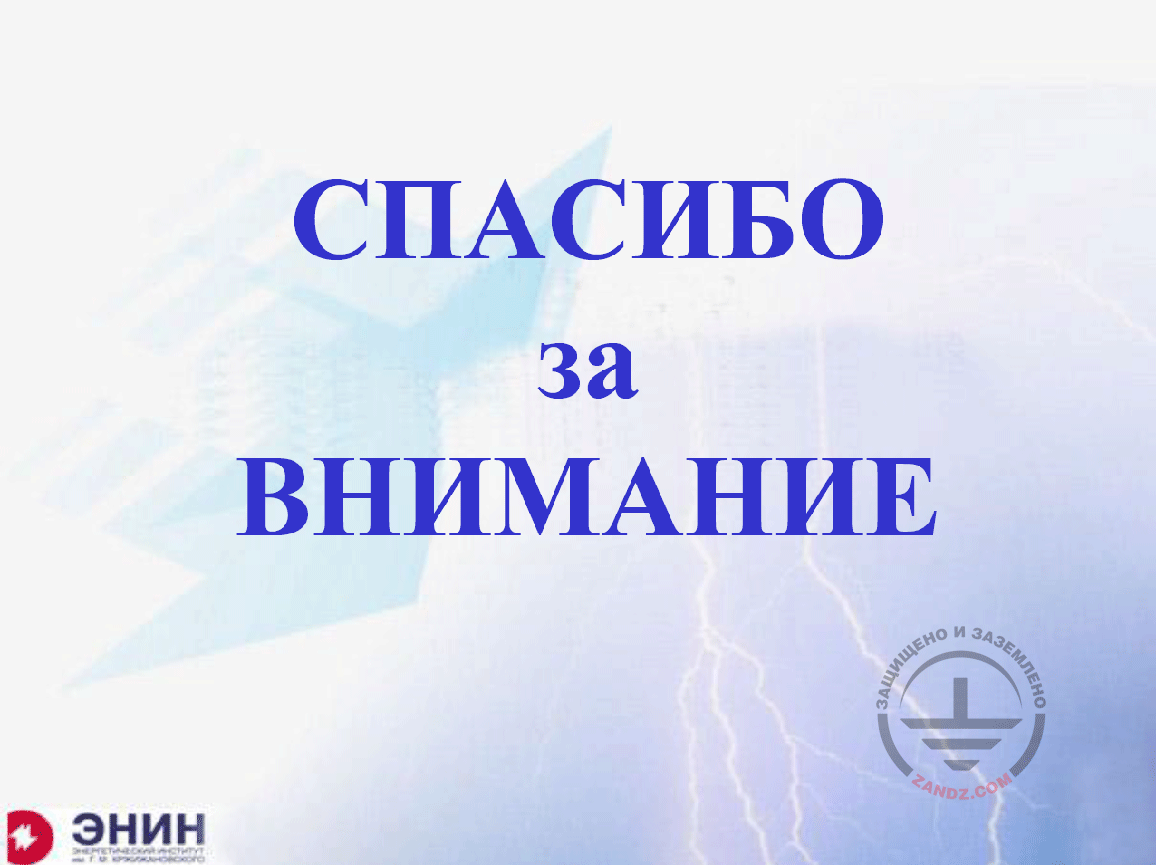Thank you for attention!

СПАСИБО ЗА ВНИМАНИЕ - THANK YOU FOR ATTENTION

<< Previous page
slides from 1 to 9

Related Articles: